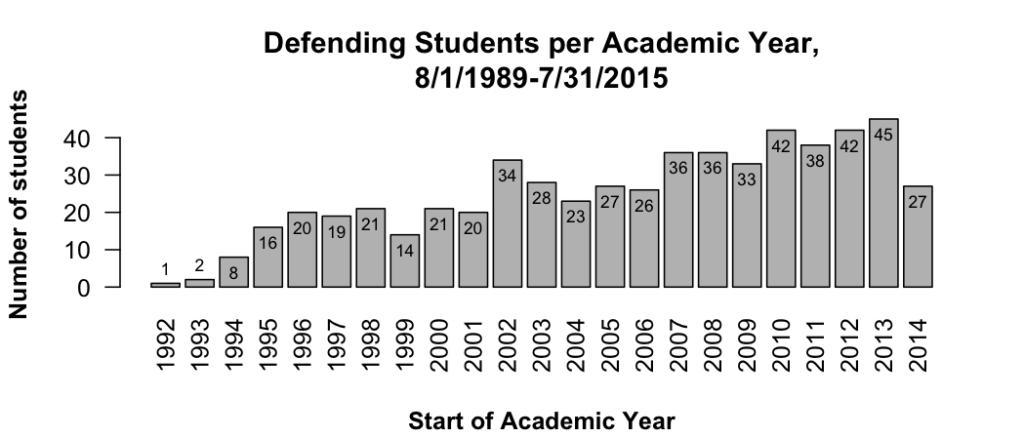# Appending Python’s Path

Find the directory that Python searchers for path additions:
`python -c 'import site; site._script()' --user-site`

In my case, it was:
~/Library/Python/2.7/lib/python/site-packages

Create a text file there with an extension “.pth” and add directories. My file looks something like:

~/Library/Python/2.7/lib/python/site-packages/mc_custom.pth:
``` # Custom additions on 5/27/16 /Users/mclaffey/Documents/my_modules ```

Source

# Non-ggplot bar graph with data labels

``` p = function() { x = gd\$year y = gd\$defended_n bp = barplot( y, names.arg = x, las = 2 # make years vertical by making all ticks perpendicular ) # title title(main="Defending Students per Academic Year,\n8/1/1989-7/31/2015") # x axis title(xlab="Start of Academic Year", font.lab=2, line=4) # y axis title(ylab="Number of students", font.lab=2) # data data labels label_height = y - 4 label_height = label_height + 8 # the first one is too low, bump it up label_height = label_height + 8 # the second one is too low, bump it up text(bp, label_height, y, cex=.7) } ```# Side-by-side bars with error bars

Simple side-by-side (no error bars):
http://stackoverflow.com/a/25070645

# Hiding the legend

``` # Remove legend for a particular aesthetic (fill) bp + guides(fill=FALSE)```

``` # It can also be done when specifying the scale bp + scale_fill_discrete(guide=FALSE) ```

```# This removes all legends bp + theme(legend.position="none") ```

# Rotating Axis Labels

http://stackoverflow.com/questions/1330989/rotating-and-spacing-axis-labels-in-ggplot2

Also makes the text smaller (assuming you are rotating because of long labels).

``` q + theme(axis.text.x = element_text(angle = 90, hjust = 1, size=20)) ```

# Percent labels for axis

http://stackoverflow.com/questions/27433798/how-to-change-y-axis-range-to-percent-from-number-in-barplot-with-r

``` library(scales) myplot <- qplot(as.factor(x), y, geom="bar") myplot + scale_y_continuous(labels=percent) ```

# Dodging

Use position='dodge' when you are okay with the default behavior.

Use position=position_dodge(width = 0.90, height=2) when you want to control the behavior.

# Zooming

If you use scale_y_XXX(limits=c(50, 100)), any graph object that falls outside that window at all will be completely removed from the figure, even if a different portion falls within the limits. To avoid this, use the following to 'zoom' in the figure, without changing what objects are included.

``` p + coord_cartesian(xlim = c(325, 500)) ```

# ggplot's text justification

http://stackoverflow.com/questions/7263849/what-do-hjust-and-vjust-do-when-making-a-plot-using-ggplot

Think of hjust as the percentage of the text's horizontal width that is left of the anchor point. So 0 means 0% of the text is left of the anchor point, so this is left justified. 0.5 means 50% of the text is left of the anchor point, so the text is right justified. 1 is 100% is right justified.

vjust is the percent of height **below** the anchor.

A way to remember this is that for an anchor point at 0,0, and with hjust=vjust=0, the text would appear properly in upper right quadrant from the origin.

Note that if the text is rotated, the justification is applied before the rotation. For example, text with hjust=0 and vjust=1 is right of and below the anchor point. If it is then rotated 90 degrees counterclockwise, it ends up right of but above the anchor point.

# Axis label spacing

The default theme doesn't have enough space between axis text and tick marks.